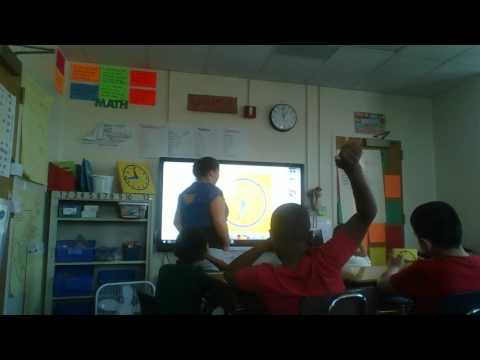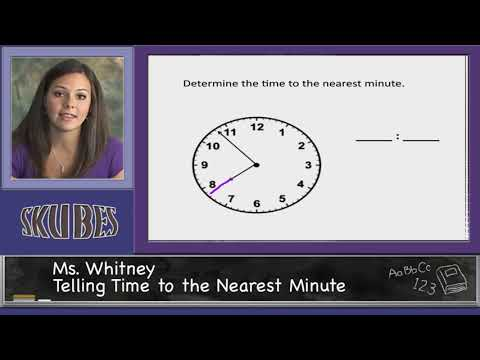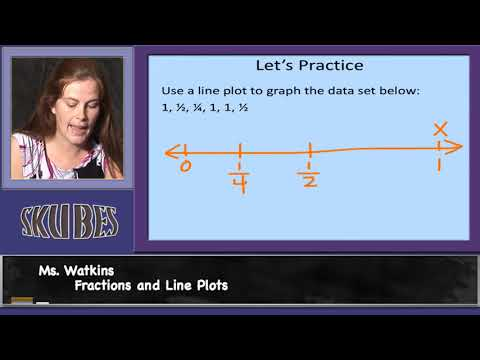# Telling Time to the Minute

##By Sabrina brown

the nearest minute and measure time intervals in minutes. Solve word problems involving addition and subtraction of time intervals in minutes# Elapsed Time on a timeline

##By Skubes ed

Tell and write time to the nearest minute and measure time intervals in minutes. Solve word problems involving addition and subtraction of time intervals in minutes# Telling Time to the Nearest Minute

##By Skubes ed

Tell and write time to the nearest minute and measure time intervals in minutes. Solve word problems involving addition and subtraction of time intervals in minutes, e.g., by representing the problem on a number line diagram.# Fractions and Line Plots

##By Skubes ed

Use the four operations to solve word problems involving distances, intervals of time, liquid volumes, masses of objects, and money, including problems involving simple fractions or decimals, and problems that require expressing measurements given in a larger unit in terms of a smaller unit.